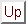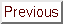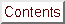Next: QR factorization Up: Annexes Previous: Newton's method for non-linear   Contents

Subsections

# Cholesky decomposition.

The Cholesky decomposition can be applied on any square matrix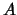which is symmetric and positive definite. The Cholesky decomposition is one of the fastest decomposition available. It constructs a lower triangular matrix L which has the following property: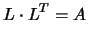(13.33)

This factorization is sometimes referred to, as taking the square root'' of the matrix.
The Cholesky decomposition is a particular case of thedecomposition. Thedecomposition is the following: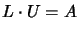(13.34)

where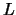is a lower triangular matrix and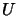is a upper triangular matrix. For example, in the case of amatrix, we have: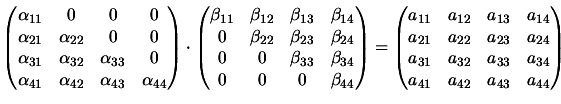(13.35)

We can use thedecomposition to solve the linear set: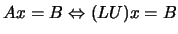by first solving for the vector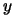such thatand then solving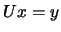. These two systems are trivial to solve because they are triangular.

## Performingdecomposition.

First let us rewrite the component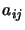offrom the equation 13.34 or 13.35. That component is always a sum beginning with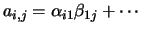The number of terms in the sum depends, however on wether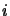or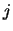is the smallest number. We have, in fact three cases: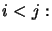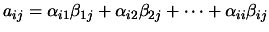(13.36)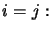(13.37)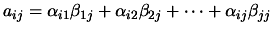(13.38)

Equations 13.36 - 13.38, totalize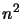equations for the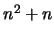unknown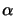's and's (the diagonal being represented twice). Since the number of unknowns is greater than the number of equations, we have to specifyof the unknowns arbitrarily and then solve for the others. In fact, as we shall see, it is always possible to take: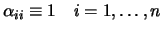(13.39)

A surprising procedure, now, is Crout's algorithm, which, quite trivially, solves the set ofEquations 13.36 - 13.38 for all the's and's by just arranging the equation in a certain order! That order is as follows:
• Set• For each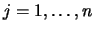do these two procedures:
First, foruse 13.36, 13.37 and 13.39 to solve for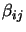, namely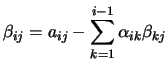(13.40)

Second, for, use 13.38 to solve for, namely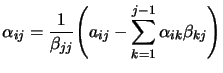(13.41)

Be sure to do both procedures before going on to the next j.
If you work through a few iterations of the above procedure, you will see that the's and's that occur on the right-hand side of the Equation 13.40 and 13.41 are already determined by the time they are needed.

## Performing Cholesky decomposition.

We can obtain the analogs of Equations 13.40 and 13.41 for the Cholesky decomposition: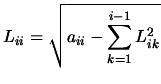(13.42)

and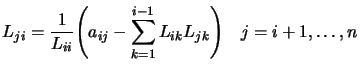(13.43)

If you apply Equation 13.42 and 13.43 in the order, you will see the the's that occur on the right-hand side are exactly determined by the time they are needed. Also, only componentswith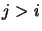are referenced.
If the matrixis not positive definite, the algorithm will stop, trying to take the square root of a negative number in equation 13.42.
What about pivoting? Pivoting (i.e., the selection of a salubrious pivot element for the division in Equation 13.43) is not really required for the stability of the algorithm. In fact, the only cause of failure is if the matrix(or, with roundoff error, another very nearby matrix) is not positive definite.Next: QR factorization Up: Annexes Previous: Newton's method for non-linear   Contents
Frank Vanden Berghen 2004-04-19